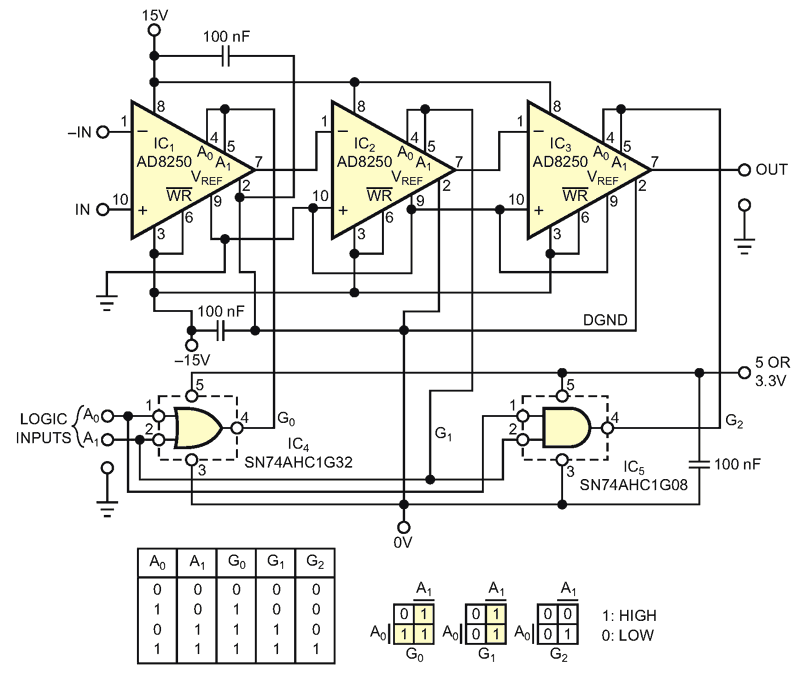# Composite instrumentation amplifier challenges single-chip device for bandwidth, offset, and noise

Although the prevailing number set in electronics is binary, human-machine interaction uses a decimal-number set. For this reason, designs often require the use of amplifiers with gain programmable in steps in the power of 10. Currently, Analog Devices’ AD8253 monolithic instrumentation amplifier is digitally programmable with voltage gains of one, 10, 100, and 1000. This IC has high bandwidth at lower gains, but you inevitably sacrifice this bandwidth when the amplifier has a gain of 1000. If your application’s demands for bandwidth reach the megahertz range at a gain of 1000 and if offset and noise performance prevail over circuit complexity, then a composite amplifier may fill the bill (Figure 1).Figure 1. Although comprising five IC packages, this digitally gain-programmable instrumentation amplifier reaches a typical bandwidth of 1.9 MHz at a gain of 1000 and thus covers the megahertz range at any of the programmable gains of one, 10, 100, and 1000.

The composite amplifier is a cascade of three Analog Devices’ AD8250 digitally gain-programmable amplifiers IC1, IC2, and IC3. The AD8250 is programmable for voltage gains of one, two, five, and 10. Because the gains of one and 10 are the only ones of interest in this case, the 2-bit words corresponding to these two values of gain are the zero and three in binary code, and the two logic pins of each of these three ICs connect. The AD8250 has a typical bandwidth of 3.8 MHz and a guaranteed bandwidth of 3 MHz at a gain of 10. The net result is that the bandwidth of the amplifier is 1.9 MHz at a gain of 1000, which is more than six times that of the single-chip AD8253. The low-frequency noise is less than 40% of that of the single-chip device.

## Materials on the topic

EDN